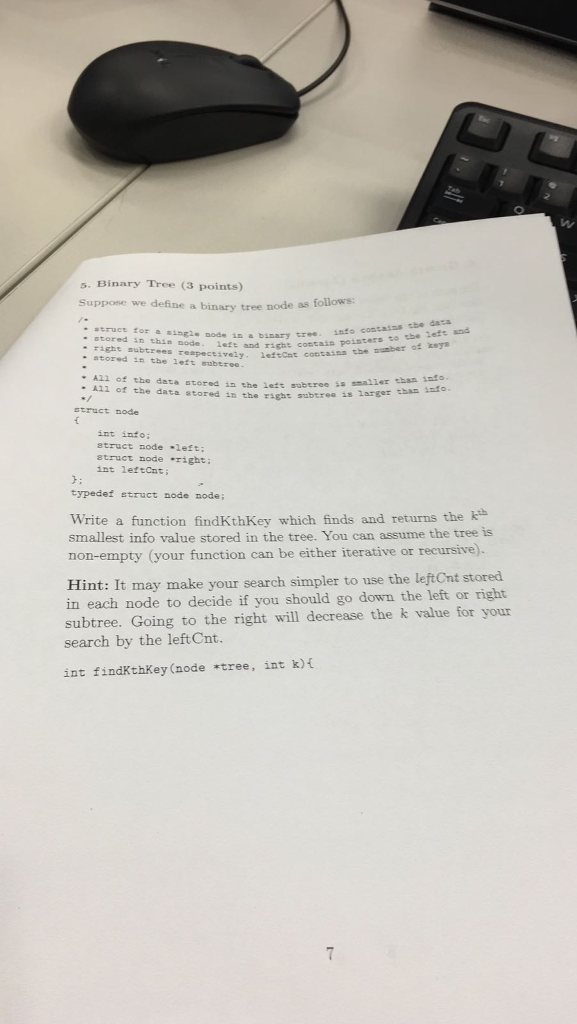# Question & Answer: Suppose we define a binary tree node as follows: /* * struct for a single node in a binary tree. info contains t…..Suppose we define a binary tree node as follows: /* * struct for a single node in a binary tree. info contains the data * stored in this node. left and right contain pointers to the left and * right subtrees respectively. leftCnt contains the number of keys * stored in the left subtree. * * All of the data stored in the left subtrees is smaller than infor. * All of the data stored in the right subtrees is larger than info. */ struct node { into info: struct node *left: struct node *right: int leftCnt: }: typedef struct node node: Write a function findKthKey which finds and returns the k^th smallest info value stored in the tree. You can assume the tree is non-empty (your function can be either iterative or recursive). int findKthKey (node *tree, int k) {

Don't use plagiarized sources. Get Your Custom Essay on
Question & Answer: Suppose we define a binary tree node as follows: /* * struct for a single node in a binary tree. info contains t…..
GET AN ESSAY WRITTEN FOR YOU FROM AS LOW AS \$13/PAGE

/*Below is the Definition of the Function you need to write*/

int findkthkey(node *tree, int k)

{
int returnValue = -1;

if( tree )
{
/* A pointer for traversal */
node *pointerTraverse = tree;

/* Find k-th smallest */
while(pointerTraverse)
{
if( (pointerTraverse->leftCnt + 1) == k )
{
returnValue = pointerTraverse->info;
break;
}
else if( k > pointerTraverse->leftCnt )
{
/* Go to right subtree If There are less no. of nodes on left subtree
*/
k = k – (pointerTraverse->leftCnt + 1);
pointerTraverse = pointerTraverse->right;
}
else
{
/* Node is on Left */
pointerTraverse = pointerTraverse->left;
}
}
}

return returnValue;
}

/*Let me know, if you face any difficulty in executing this*/